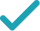Type a math problemSolve for xSteps for Solving Linear Equation
Factor . Rewrite the square root of the product as the product of square roots . Take the square root of .
Assign xGraphGraph Both Sides in 2D
Graph in 2DGiving is as easy as 1, 2, 3
Get 1,000 points to donate to a school of your choice when you join Give With Bing
x=-\frac{4}{3}+2\sqrt{13}
Factor 52=2^{2}\times 13. Rewrite the square root of the product \sqrt{2^{2}\times 13}\approx 7.211102551 as the product of square roots \sqrt{2^{2}}\sqrt{13}\approx 7.211102551. Take the square root of 2^{2}\approx 4.